|

# 买了就后悔的6种椅子

关于教消费者如何挑选一张舒适座椅的文章已经相当多了，选择什么样的座椅更是萝卜青菜各有所爱，不能强求。但是以下6种座椅在人体工程学设计或安全方面有缺陷，久坐后对身体的损坏较大，小编总结出来，供您参考。

1、气棒来历不明，未通过安全认证的座椅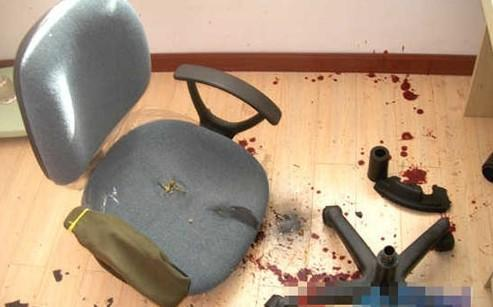2007年4月浙江省舟山市娄女士坐的椅子发生爆炸，硬塑料、木屑、海绵、布料、密封圈等大量碎片崩进娄女士体内。

2007年11月26日，烟台开发区68岁的吴老先生的椅子发生爆炸，臀部、腿部、直肠肛管和小肠受伤严重。

2009年12月，家住山东胶州新立街小区的14岁男孩涛涛像往常一样，坐着旋转升降椅子上网，却没想发生意外，旋转椅突然发生爆炸，椅子气压杆直直插进了涛涛的臀部，胸腔被一块小碎片击穿，致其呼吸功能衰竭死亡。

2012年8月11日晚9点45分，居住在厦门的刘小姐像往常一样，洗完澡，坐在电脑桌前的气压升降椅上，吹头发。突然砰一声巨响，椅子就炸开了。刘小姐当即摔在地上，弟弟将其送往医院。医生从她腹腔和臀部取出几十块铁环、螺丝和碎片。

一篇题为"旋转椅杀人，你坐什么样的椅子？"的帖子在网上引起轩然大波。网友表态：“我们可以忍受不舒适的椅子，但是绝不可以忍受伤人的椅子！”这一切悲剧的根源就是这些无品牌或杂牌的座椅使用了无国家和行业安全认证的劣质气压棒。

所以您在选购座椅时，首先还是建议选择一些知名品牌较为安全，其次就要看得气压棒是否安全。您可以先检查气压棒，一般正规气压棒会在棒身上刻有品牌logo和相关参数，再询问销售员气压棒是哪个工厂生产的，有没有通过ISO9001国家安全质量认证或SGS安全认证，并让他出示相关的证明文件。第三，您要看座椅下是否有2-4毫米厚的整块防爆钢板，即使万一气棒爆炸，也有一层安全防护。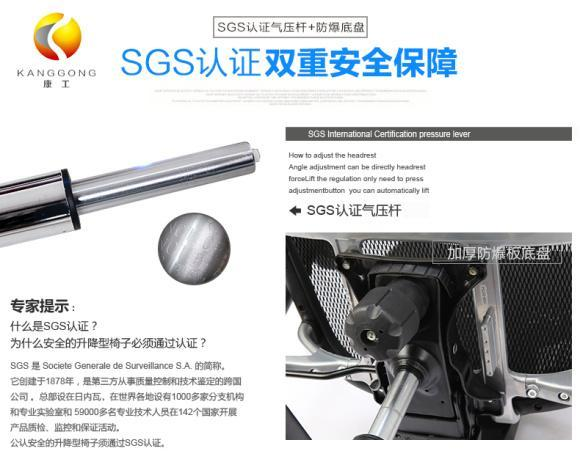2、坐垫、背垫为皮制或海棉的座椅

在传统的观念中，高档座椅就是要真皮的，豪华大气；次一点的是海棉+布料，坐得也软乎。您购买这类材质的座椅也没有错，唯一不足的就是在炎热的夏季，坐在这类座椅上就像坐在暖炉上一样，既不透气也不排汗，有的久坐的人屁股上还长了痱子。这类材质时间久了还容易滋生螨虫。

随着新材料的发展，在全球范围内，高强度网布替代皮、棉布和海棉成为座椅的主流材质。高档网布不仅耐磨、耐压、承重力强，而且易清洗、透气性好，坐感舒适，避免由于久坐臀部腿部过热产生的不适感，是您久坐选择的最佳座椅材质。

有人担心网布如果被烟头烫了洞，会不会整个就报废了？网布也分三六九等，小编告诉您一个挑选网布座椅的简单易行的实验方法。您向销售员要一块坐垫网布的样品，在征得销售员同意的情况下，用烟头在上面烫个洞，然后在洞口处用力撕扯这块网布，如果是高质量的网布，即使您烫了一个洞，洞周围的网线连接仍然很牢固，即使撕扯也没有断开的迹象。而如果是劣质网布，一撕扯整个网布就断开报废了。有人担心网布材质的座椅是不是很贵？小编可以负责任的说，一些优秀品牌的办公椅，例如康工办公椅，几百元也可以买到高档的全网布座椅。3、靠不到椅背的座椅这类座椅是最坑爹的，坐垫已经坐满了，可是坐垫太长，后背还是靠不到椅背上，不得已只得斜靠在椅背上，不出半个小时，肯定腰疼。

所以在挑选座椅时，您一定要试坐一下，坐垫的长度要以前端达到膝窝处，后背正好稳稳的靠在椅背上为宜，让坐垫尽量多的接触臀部和大腿的面积，增大接触面积，减少单位压强，身体才能久坐不累。

如果座椅带有坐垫深度调节功能就更好了，您可以根据自己的腿长调节坐垫深度，让自己坐得更加舒适。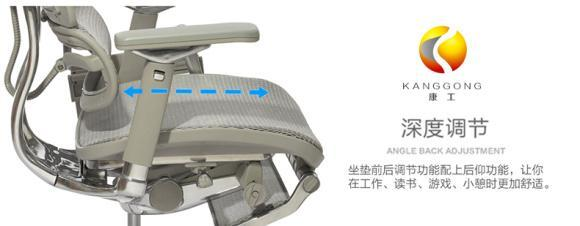4、没有腰枕的座椅

很多人都忽视了腰枕在座椅中的重要性。人体的脊椎是个自然的S型，在腰部的脊椎是向前突出的，如果在坐的时候没有合适的腰枕顶着腰椎，即人体脊椎从下向上数第二、三、四节脊柱骨的位置，人们会不由自主的弯腰驼背，久而久之，腰酸背痛，脊椎病变就开始找上门来。

所以腰枕对座椅的健康性不言而喻，只有它顶在用户的腰椎处，用户的上身躯干才能自然挺直，打开胸腔，呼吸顺畅，提高工作效率，同时避免不正确坐姿对脊椎上半部分的损害。

一些高档的人体工程学座椅，例如康工电脑椅等，不仅腰枕的高度可以调节，而且还有弹性，使得使用者的背部稍离开椅背一点或者左右转身时，腰部都能得到腰枕的有力支撑。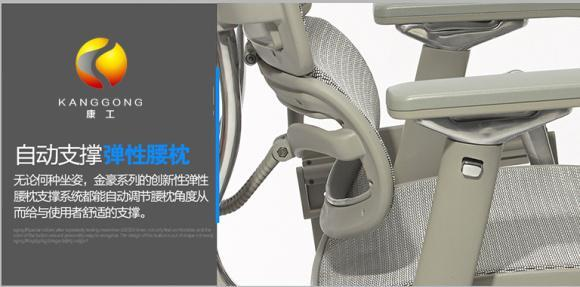5、没有后仰功能的座椅

久坐导致腰椎颈椎病的案例屡见不鲜。腰椎颈椎病的大规模出现一方面与久坐有关，另一方面与错误的坐姿有关。传统的坐姿告诉我们要坐端正，实际上，现代的科学研究证明，倾斜角度大约达到135°时的半躺式坐姿对人体的伤害最小，是比笔直坐姿更健康的坐姿。

最近，在北美一年一度的放射线学会上，英国一个由放射线学者们组成的小组公布了在英国阿伯丁市伍登德医院进行的坐姿测试结果。参加测试的人都是没有患过背部疼痛、也没有进行过外科手术的志愿者。在测试期间，每个志愿者可以同在日常生活中一样自由地行动，摆出各种姿势，但是，他们必须完成几个规定动作，分别是以随意地前倾、90度和135度三种坐姿坐下。

在做这三个规定动作的过程中，研究人员一直用一台移动的磁力共振机器扫描他们的脊柱，观察他们的脊柱在变换角度时的状态，然后，研究人员对仪器的记录结果进行了分析。

研究小组负责人魏塞姆·阿廖尔·巴塞尔博士说：“通过对仪器扫描结果的分析，我们发现，与弯腰驼背或者向前倾的坐姿相比，90度角坐姿的确更胜一筹。但是在笔直坐姿之下，椎间盘发出的声响依然很大，同时磨损率也很高。因为几乎整个上半身的重量都作用于脊椎骨的最下面两个部分——腰椎和骶骨。如果坐得笔直，脊椎会被拉得很紧，连带脊椎周围的肌肉和韧带也会被拉紧，时间长了就会引起慢性疼痛。而后仰的坐姿，特别是135度的后仰坐姿是最有利于保持脊柱的形状，保持脊柱健康的。”

巴塞尔博士强调，从实验中发现，以135°后倾角度坐着，作用在椎间盘和相连肌肉和肌腱上的压力最小，体重被更平均地分配在整条脊椎骨上。不但使身体可以自然放松而且对脊椎的压力可以减少到最小。如果椅背固定90度，而强行在椅子里坐135度姿势，对背部和脊椎的伤害同样不小。135度坐姿也讲究细节。背部必须保持紧贴椅背，确保脊柱有受力点支撑。这意味着，一张能够调节靠背角度的椅子必不可少。

所以为了脊椎长久的健康，你必须学会135度的健康坐姿，在挑选座椅时，也要挑选能够后仰并可锁定的座椅。6、不带头枕的座椅

现代人很多有头痛、背痛、手麻的情况，这些多半跟颈椎病变有关。人们长时间久坐，脖子保持一个姿势看屏幕，时间久了，脖子不堪重负，发生病变也是情理之中。要减轻脖子的负担，除休息运动之外，座椅头枕的作用就突显出来。

在135度健康坐姿中，座椅的头枕可以承担头部的重量，减轻颈部的压力，有效防止颈椎病的发生。一些人体工学电脑椅品牌，如康工等，不仅座椅配置头枕，而且头枕可调节高度和角度，被称之为2D头枕，让使用者获得更为舒适的坐感体验。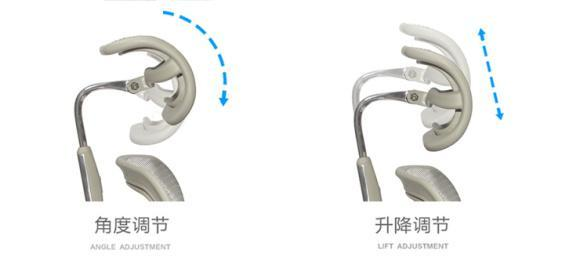选择什么样的座椅是您的权利，但是如果遇到上述6种座椅，请思考下使用过程中会遇到的种种问题，结合自身的实际需求，货比三家后再做决定。

传统座椅向人体工程学座椅演变已经是大势所趋，现代人们不仅要坐得舒适，更要坐得健康，对自己的身体负责。康工是人体工学电脑椅的领军品牌之一，定位于为客户提供健康办公解决方案，让客户在工作中享受健康。康工电脑椅充分考虑到用户的舒适和健康，精心设计，拥有十几项个性化的调节功能，是众多世界500强公司办公用椅的首选，也是您的明智之选。`声明：本文由入驻焦点开放平台的作者撰写，除焦点官方账号外，观点仅代表作者本人，不代表焦点立场错误信息举报电话： 400-099-0099，邮箱：jubao@vip.sohu.com，或点此进行意见反馈，或点此进行举报投诉。`A B C D E F G H J K L M N P Q R S T W X Y Z
A - B - C - D - E
• A
• 鞍山
• 安庆
• 安阳
• 安顺
• 安康
• 澳门
• B
• 北京
• 保定
• 包头
• 巴彦淖尔
• 本溪
• 蚌埠
• 亳州
• 滨州
• 北海
• 百色
• 巴中
• 毕节
• 保山
• 宝鸡
• 白银
• 巴州
• C
• 承德
• 沧州
• 长治
• 赤峰
• 朝阳
• 长春
• 常州
• 滁州
• 池州
• 长沙
• 常德
• 郴州
• 潮州
• 崇左
• 重庆
• 成都
• 楚雄
• 昌都
• 慈溪
• 常熟
• D
• 大同
• 大连
• 丹东
• 大庆
• 东营
• 德州
• 东莞
• 德阳
• 达州
• 大理
• 德宏
• 定西
• 儋州
• 东平
• E
• 鄂尔多斯
• 鄂州
• 恩施
F - G - H - I - J
• F
• 抚顺
• 阜新
• 阜阳
• 福州
• 抚州
• 佛山
• 防城港
• G
• 赣州
• 广州
• 桂林
• 贵港
• 广元
• 广安
• 贵阳
• 固原
• H
• 邯郸
• 衡水
• 呼和浩特
• 呼伦贝尔
• 葫芦岛
• 哈尔滨
• 黑河
• 淮安
• 杭州
• 湖州
• 合肥
• 淮南
• 淮北
• 黄山
• 菏泽
• 鹤壁
• 黄石
• 黄冈
• 衡阳
• 怀化
• 惠州
• 河源
• 贺州
• 河池
• 海口
• 红河
• 汉中
• 海东
• 怀来
• I
• J
• 晋中
• 锦州
• 吉林
• 鸡西
• 佳木斯
• 嘉兴
• 金华
• 景德镇
• 九江
• 吉安
• 济南
• 济宁
• 焦作
• 荆门
• 荆州
• 江门
• 揭阳
• 金昌
• 酒泉
• 嘉峪关
K - L - M - N - P
• K
• 开封
• 昆明
• 昆山
• L
• 廊坊
• 临汾
• 辽阳
• 连云港
• 丽水
• 六安
• 龙岩
• 莱芜
• 临沂
• 聊城
• 洛阳
• 漯河
• 娄底
• 柳州
• 来宾
• 泸州
• 乐山
• 六盘水
• 丽江
• 临沧
• 拉萨
• 林芝
• 兰州
• 陇南
• M
• 牡丹江
• 马鞍山
• 茂名
• 梅州
• 绵阳
• 眉山
• N
• 南京
• 南通
• 宁波
• 南平
• 宁德
• 南昌
• 南阳
• 南宁
• 内江
• 南充
• P
• 盘锦
• 莆田
• 平顶山
• 濮阳
• 攀枝花
• 普洱
• 平凉
Q - R - S - T - W
• Q
• 秦皇岛
• 齐齐哈尔
• 衢州
• 泉州
• 青岛
• 清远
• 钦州
• 黔南
• 曲靖
• 庆阳
• R
• 日照
• 日喀则
• S
• 石家庄
• 沈阳
• 双鸭山
• 绥化
• 上海
• 苏州
• 宿迁
• 绍兴
• 宿州
• 三明
• 上饶
• 三门峡
• 商丘
• 十堰
• 随州
• 邵阳
• 韶关
• 深圳
• 汕头
• 汕尾
• 三亚
• 三沙
• 遂宁
• 山南
• 商洛
• 石嘴山
• T
• 天津
• 唐山
• 太原
• 通辽
• 铁岭
• 泰州
• 台州
• 铜陵
• 泰安
• 铜仁
• 铜川
• 天水
• 天门
• W
• 乌海
• 乌兰察布
• 无锡
• 温州
• 芜湖
• 潍坊
• 威海
• 武汉
• 梧州
• 渭南
• 武威
• 吴忠
• 乌鲁木齐
X - Y - Z
• X
• 邢台
• 徐州
• 宣城
• 厦门
• 新乡
• 许昌
• 信阳
• 襄阳
• 孝感
• 咸宁
• 湘潭
• 湘西
• 西双版纳
• 西安
• 咸阳
• 西宁
• 仙桃
• 西昌
• Y
• 运城
• 营口
• 盐城
• 扬州
• 鹰潭
• 宜春
• 烟台
• 宜昌
• 岳阳
• 益阳
• 永州
• 阳江
• 云浮
• 玉林
• 宜宾
• 雅安
• 玉溪
• 延安
• 榆林
• 银川
• Z
• 张家口
• 镇江
• 舟山
• 漳州
• 淄博
• 枣庄
• 郑州
• 周口
• 驻马店
• 株洲
• 张家界
• 珠海
• 湛江
• 肇庆
• 中山
• 自贡
• 资阳
• 遵义
• 昭通
• 张掖
• 中卫

1室1厅1厨1卫1阳台

1
2
3
4
5

0
1
2

1

1

0
1
2
3报名成功，资料已提交审核A B C D E F G H J K L M N P Q R S T W X Y Z
A - B - C - D - E
• A
• 鞍山
• 安庆
• 安阳
• 安顺
• 安康
• 澳门
• B
• 北京
• 保定
• 包头
• 巴彦淖尔
• 本溪
• 蚌埠
• 亳州
• 滨州
• 北海
• 百色
• 巴中
• 毕节
• 保山
• 宝鸡
• 白银
• 巴州
• C
• 承德
• 沧州
• 长治
• 赤峰
• 朝阳
• 长春
• 常州
• 滁州
• 池州
• 长沙
• 常德
• 郴州
• 潮州
• 崇左
• 重庆
• 成都
• 楚雄
• 昌都
• 慈溪
• 常熟
• D
• 大同
• 大连
• 丹东
• 大庆
• 东营
• 德州
• 东莞
• 德阳
• 达州
• 大理
• 德宏
• 定西
• 儋州
• 东平
• E
• 鄂尔多斯
• 鄂州
• 恩施
F - G - H - I - J
• F
• 抚顺
• 阜新
• 阜阳
• 福州
• 抚州
• 佛山
• 防城港
• G
• 赣州
• 广州
• 桂林
• 贵港
• 广元
• 广安
• 贵阳
• 固原
• H
• 邯郸
• 衡水
• 呼和浩特
• 呼伦贝尔
• 葫芦岛
• 哈尔滨
• 黑河
• 淮安
• 杭州
• 湖州
• 合肥
• 淮南
• 淮北
• 黄山
• 菏泽
• 鹤壁
• 黄石
• 黄冈
• 衡阳
• 怀化
• 惠州
• 河源
• 贺州
• 河池
• 海口
• 红河
• 汉中
• 海东
• 怀来
• I
• J
• 晋中
• 锦州
• 吉林
• 鸡西
• 佳木斯
• 嘉兴
• 金华
• 景德镇
• 九江
• 吉安
• 济南
• 济宁
• 焦作
• 荆门
• 荆州
• 江门
• 揭阳
• 金昌
• 酒泉
• 嘉峪关
K - L - M - N - P
• K
• 开封
• 昆明
• 昆山
• L
• 廊坊
• 临汾
• 辽阳
• 连云港
• 丽水
• 六安
• 龙岩
• 莱芜
• 临沂
• 聊城
• 洛阳
• 漯河
• 娄底
• 柳州
• 来宾
• 泸州
• 乐山
• 六盘水
• 丽江
• 临沧
• 拉萨
• 林芝
• 兰州
• 陇南
• M
• 牡丹江
• 马鞍山
• 茂名
• 梅州
• 绵阳
• 眉山
• N
• 南京
• 南通
• 宁波
• 南平
• 宁德
• 南昌
• 南阳
• 南宁
• 内江
• 南充
• P
• 盘锦
• 莆田
• 平顶山
• 濮阳
• 攀枝花
• 普洱
• 平凉
Q - R - S - T - W
• Q
• 秦皇岛
• 齐齐哈尔
• 衢州
• 泉州
• 青岛
• 清远
• 钦州
• 黔南
• 曲靖
• 庆阳
• R
• 日照
• 日喀则
• S
• 石家庄
• 沈阳
• 双鸭山
• 绥化
• 上海
• 苏州
• 宿迁
• 绍兴
• 宿州
• 三明
• 上饶
• 三门峡
• 商丘
• 十堰
• 随州
• 邵阳
• 韶关
• 深圳
• 汕头
• 汕尾
• 三亚
• 三沙
• 遂宁
• 山南
• 商洛
• 石嘴山
• T
• 天津
• 唐山
• 太原
• 通辽
• 铁岭
• 泰州
• 台州
• 铜陵
• 泰安
• 铜仁
• 铜川
• 天水
• 天门
• W
• 乌海
• 乌兰察布
• 无锡
• 温州
• 芜湖
• 潍坊
• 威海
• 武汉
• 梧州
• 渭南
• 武威
• 吴忠
• 乌鲁木齐
X - Y - Z
• X
• 邢台
• 徐州
• 宣城
• 厦门
• 新乡
• 许昌
• 信阳
• 襄阳
• 孝感
• 咸宁
• 湘潭
• 湘西
• 西双版纳
• 西安
• 咸阳
• 西宁
• 仙桃
• 西昌
• Y
• 运城
• 营口
• 盐城
• 扬州
• 鹰潭
• 宜春
• 烟台
• 宜昌
• 岳阳
• 益阳
• 永州
• 阳江
• 云浮
• 玉林
• 宜宾
• 雅安
• 玉溪
• 延安
• 榆林
• 银川
• Z
• 张家口
• 镇江
• 舟山
• 漳州
• 淄博
• 枣庄
• 郑州
• 周口
• 驻马店
• 株洲
• 张家界
• 珠海
• 湛江
• 肇庆
• 中山
• 自贡
• 资阳
• 遵义
• 昭通
• 张掖
• 中卫• 手机• 分享
• 设计
免费设计
• 计算器
装修计算器
• 入驻
合作入驻
• 联系
联系我们
• 置顶
返回顶部